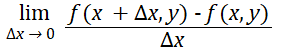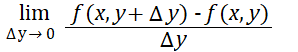# Partial Derivative

A partial derivative is a derivative where one or more variables is held constant.

When you have a multivariate function with more than one independent variable, like z = f (x, y), both variables x and y can affect z. The partial derivative holds one variable constant, allowing you to investigate how a small change in the second variable affects the function’s output. For example, the partial derivative of z with respect to x holds y constant. Essentially, you find the derivative for just one of the function’s variables.

Formally, the partial derivative for a single-valued function z = f(x, y) is defined for z with respect to x (i.e. where y is held constant) as:And for z with respect to y (where x is held constant) as:With univariate functions, there’s only one variable, so the partial derivative and ordinary derivative are conceptually the same (De la Fuente, 2000).

## Notation

A partial derivative can be denoted in many different ways.

A common way is to use subscripts to show which variable is being differentiated. For example, Dxi f(x), fxi(x), fi(x) or fx.

Which notation you use depends on the preference of the author, instructor, or the particular field you’re working in. For example, in thermodynamics, (∂z.∂xi)x ≠ xi (with curly d notation) is standard for the partial derivative of a function z = (xi,…, xn) with respect to xi (Sychev, 1991).

## How To Find a Partial Derivative: Example

You find partial derivatives in the same way as ordinary derivatives (e.g. with the chain rule or product rule. The only difference is that before you find the derivative for one variable, you must hold the other constant.

Example Question: Find the partial derivative of the following function with respect to x:
f(x, y) = x2 + y4.

Step 1: Change the variable you’re not differentiating to a constant. It doesn’t matter which constant you choose, because all constants have a derivative of zero. For this question, you’re differentiating with respect to x, so I’m going to put an arbitrary “10” in as the constant:
f(x, y) = x2 + 10.

Step 2: Differentiate as usual. For this particular function, use the power rule:
f′x = 2x(2-1) + 0 = 2x.

The partial derivative for this function with respect to x is 2x.

## References

Abramowitz, M. and Stegun, I. A. (Eds.). Handbook of Mathematical Functions with Formulas, Graphs, and Mathematical Tables, 9th printing. New York: Dover, pp. 883-885, 1972.
De la Fuente, A. (2000). Mathematical Methods and Models for Economists. Cambridge University Press.
Sychev, V. (1991). The Differential Equations Of Thermodynamics. CRC Press.
Thomas, G. B. and Finney, R. L. §16.8 in Calculus and Analytic Geometry, 9th ed. Reading, MA: Addison-Wesley, 1996.

CITE THIS AS:
Stephanie Glen. "Partial Derivative" From StatisticsHowTo.com: Elementary Statistics for the rest of us! https://www.statisticshowto.com/partial-derivative/